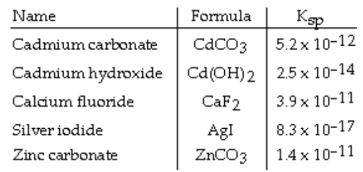# Problem: Consider the following table of Ksp values.Which compound listed below has the smallest molar solubility in water?

###### FREE Expert Solution

We’re being asked to determine which compound has the smallest molar solubility in pure water.

Recall that Ksp is an equilibrium constant related to slightly soluble ionic compounds

The dissociation of slightly soluble ionic compounds in solution is as follows:

MnNm(s)  n Mm+(aq) + m Nn–(aq)

We can construct an ICE table for this dissociation

Remember that solids are ignored in the ICE table and Ksp expression.

90% (213 ratings)###### Problem Details

Consider the following table of Ksp values.Which compound listed below has the smallest molar solubility in water?